# Thermodynamics Laws of thermodynamics Annapoorna M Introduction Thermodynamics

• Slides: 20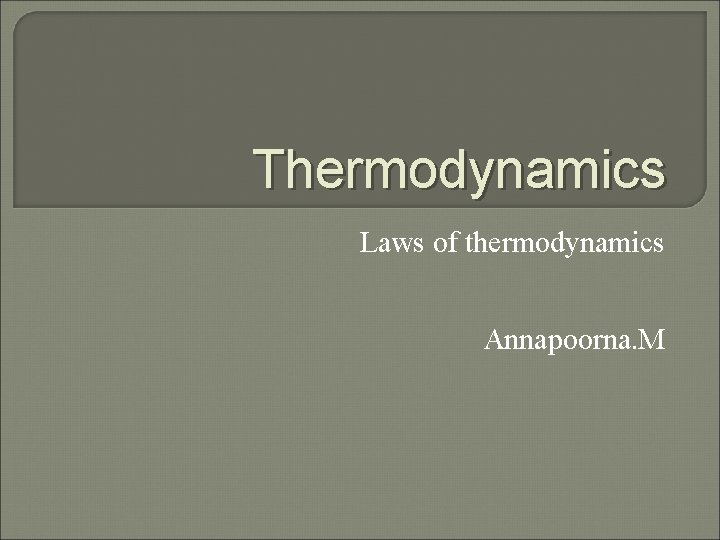Thermodynamics Laws of thermodynamics Annapoorna. M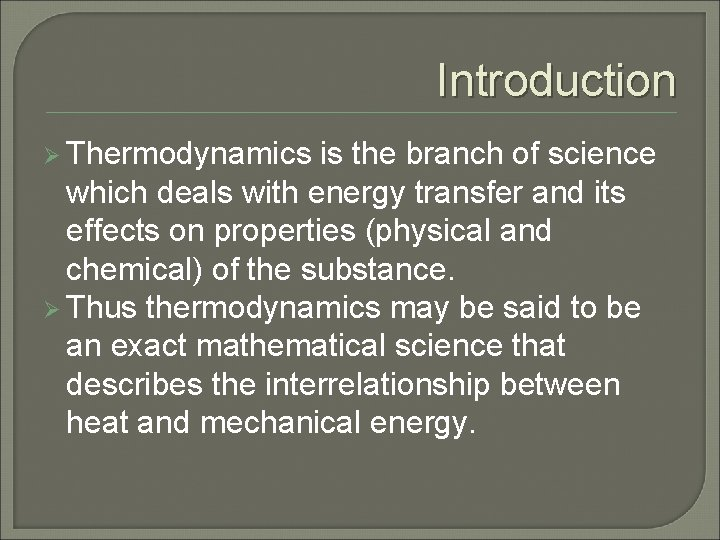Introduction Ø Thermodynamics is the branch of science which deals with energy transfer and its effects on properties (physical and chemical) of the substance. Ø Thus thermodynamics may be said to be an exact mathematical science that describes the interrelationship between heat and mechanical energy.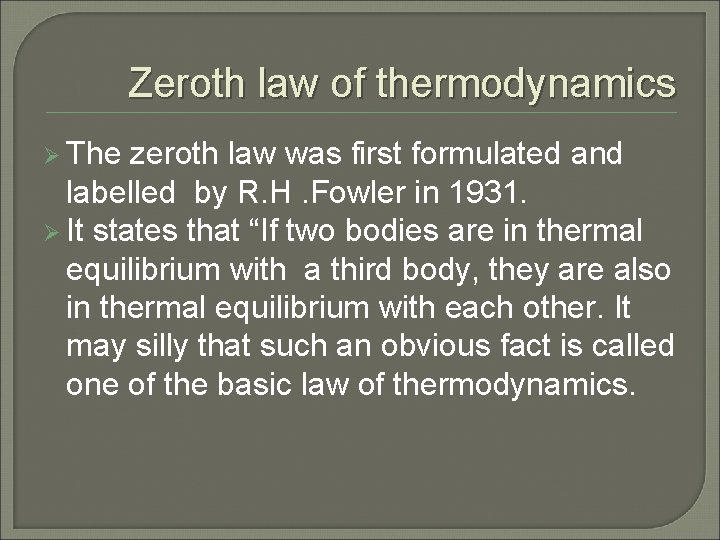Zeroth law of thermodynamics Ø The zeroth law was first formulated and labelled by R. H. Fowler in 1931. Ø It states that “If two bodies are in thermal equilibrium with a third body, they are also in thermal equilibrium with each other. It may silly that such an obvious fact is called one of the basic law of thermodynamics.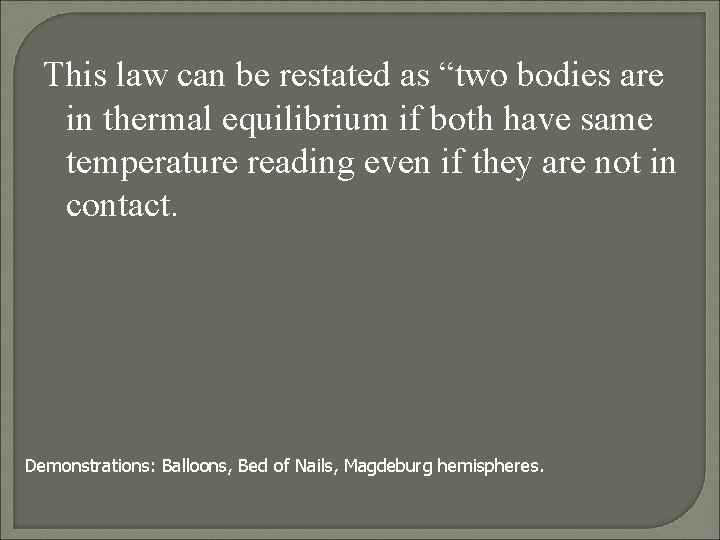This law can be restated as “two bodies are in thermal equilibrium if both have same temperature reading even if they are not in contact. Demonstrations: Balloons, Bed of Nails, Magdeburg hemispheres.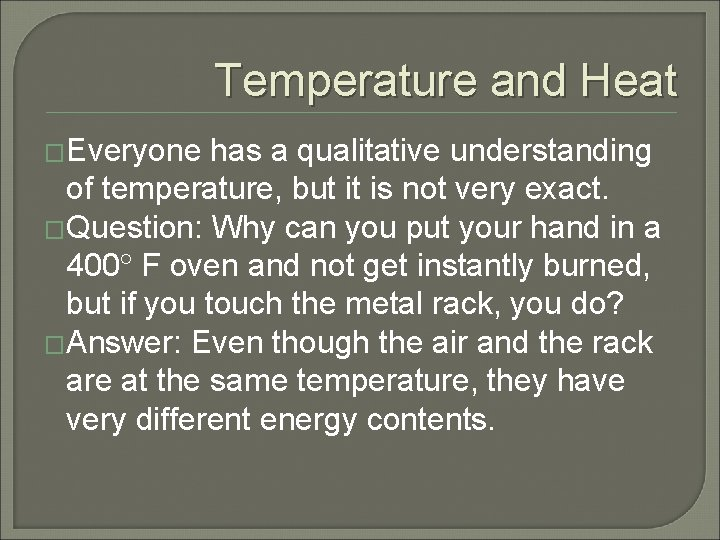Temperature and Heat �Everyone has a qualitative understanding of temperature, but it is not very exact. �Question: Why can you put your hand in a 400 F oven and not get instantly burned, but if you touch the metal rack, you do? �Answer: Even though the air and the rack are at the same temperature, they have very different energy contents.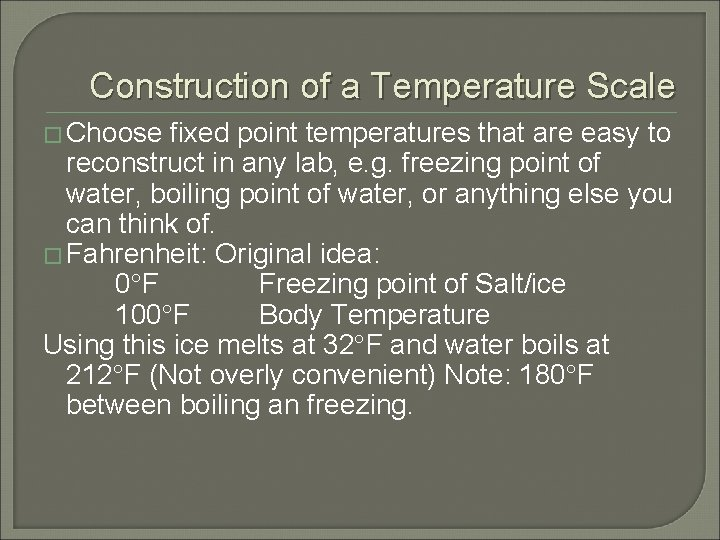Construction of a Temperature Scale � Choose fixed point temperatures that are easy to reconstruct in any lab, e. g. freezing point of water, boiling point of water, or anything else you can think of. � Fahrenheit: Original idea: 0 F Freezing point of Salt/ice 100 F Body Temperature Using this ice melts at 32 F and water boils at 212 F (Not overly convenient) Note: 180 F between boiling an freezing.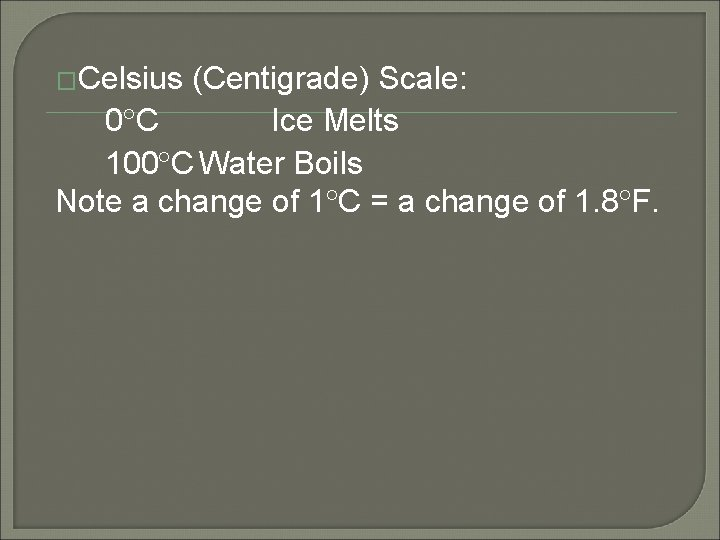�Celsius (Centigrade) Scale: 0 C Ice Melts 100 C Water Boils Note a change of 1 C = a change of 1. 8 F.First Law of Thermodynamics Conservation of energy �When heat is added into a system it can either 1) change the internal energy of the system (i. e. make it hotter) or 2) go into doing work. Q=W + U. Note: For our purposes, Internal Energy is the part of the energy that depends on Temperature.Heat Engines �If we can create an “engine” that operates in a cycle, we return to our starting point each time and therefore have the same internal energy. Thus, for a complete cycle Q=W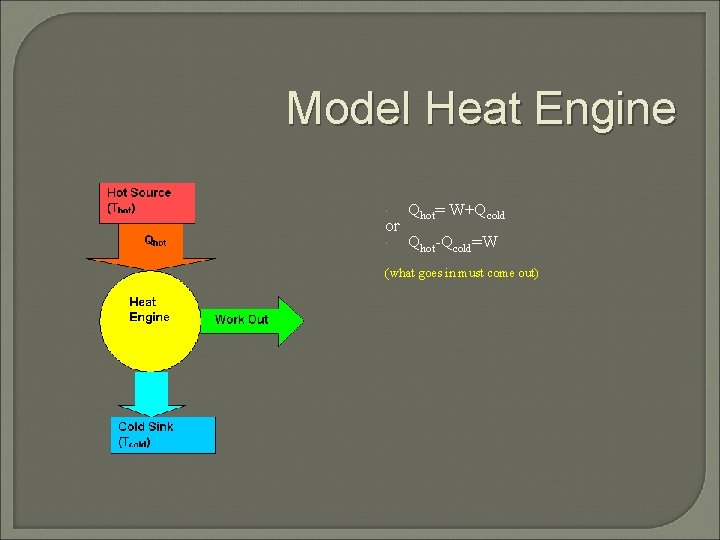Model Heat Engine Qhot= W+Qcold Qhot-Qcold=W or (what goes in must come out)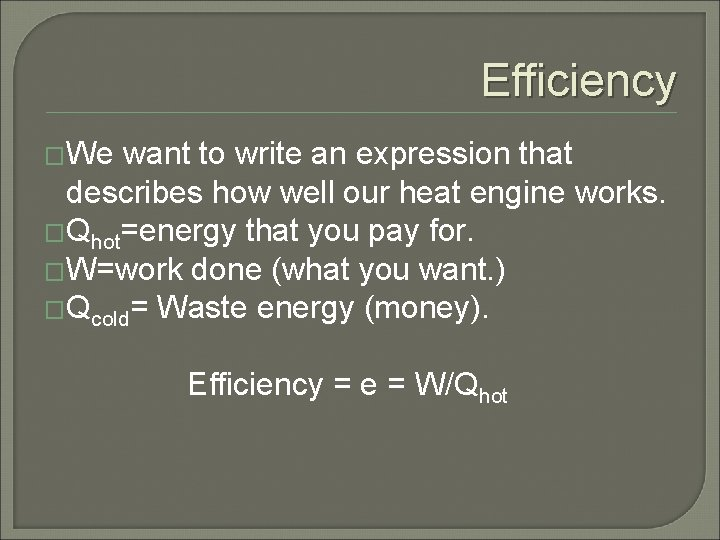Efficiency �We want to write an expression that describes how well our heat engine works. �Qhot=energy that you pay for. �W=work done (what you want. ) �Qcold= Waste energy (money). Efficiency = e = W/Qhot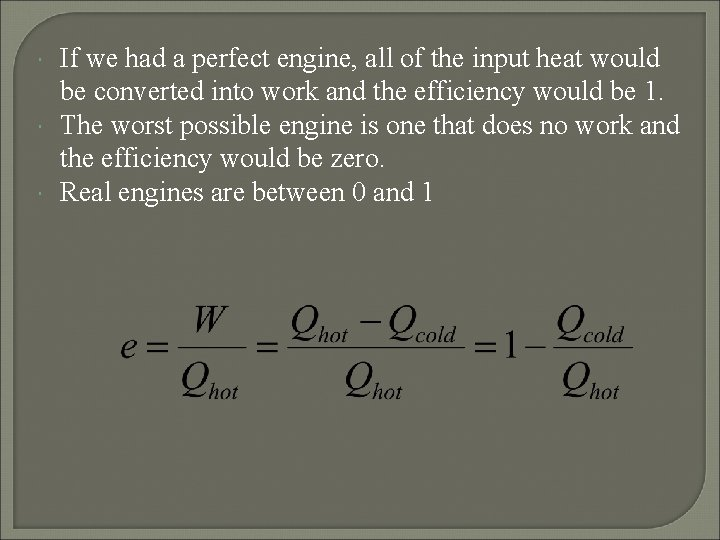If we had a perfect engine, all of the input heat would be converted into work and the efficiency would be 1. The worst possible engine is one that does no work and the efficiency would be zero. Real engines are between 0 and 1Newcomen Engine (First real steam engine) e=0. 005Example Calculation �In every cycle, a heat engine absorbs 1000 J from a hot reservoir at 600 K, does 400 J of work and expels 600 J into a cold reservoir at 300 K. Calculate the efficiency of the engine. �e= 400 J/1000 J=0. 4 �This is actually a pretty good engine.Second Law of Thermodynamics (What can actually happen) �Heat does not voluntarily flow from cold to hot. OR �All heat engines have e<1. (Not all heat can be converted into work. )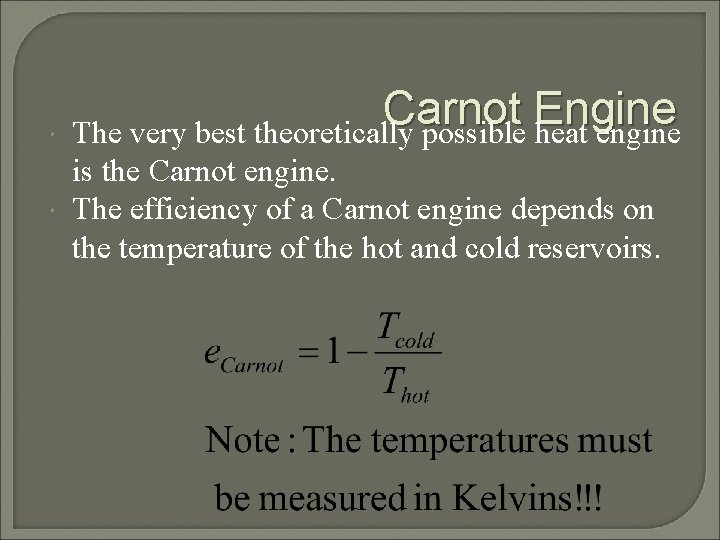Carnot Engine The very best theoretically possible heat engine is the Carnot engine. The efficiency of a Carnot engine depends on the temperature of the hot and cold reservoirs.Example Calculation Part II �In every cycle, a heat engine absorbs 1000 J from a hot reservoir at 600 K, does 400 J of work and expels 600 J into a cold reservoir at 300 K. Calculate the efficiency of the best possible engine. �e= 1 -300/600 =0. 5 �Recall that the actual engine has e=0. 4.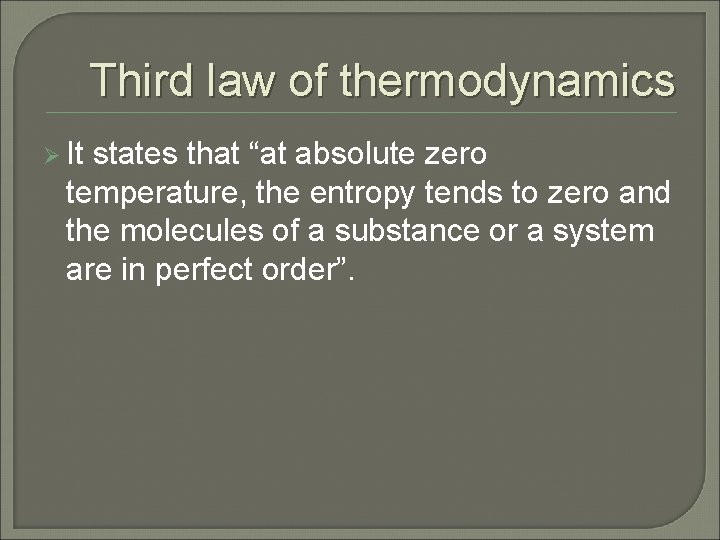Third law of thermodynamics Ø It states that “at absolute zero temperature, the entropy tends to zero and the molecules of a substance or a system are in perfect order”.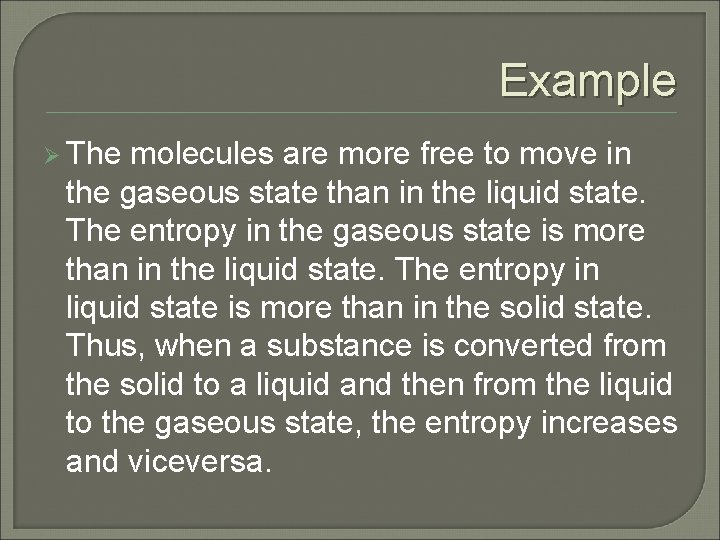Example Ø The molecules are more free to move in the gaseous state than in the liquid state. The entropy in the gaseous state is more than in the liquid state. The entropy in liquid state is more than in the solid state. Thus, when a substance is converted from the solid to a liquid and then from the liquid to the gaseous state, the entropy increases and viceversa.Thank you